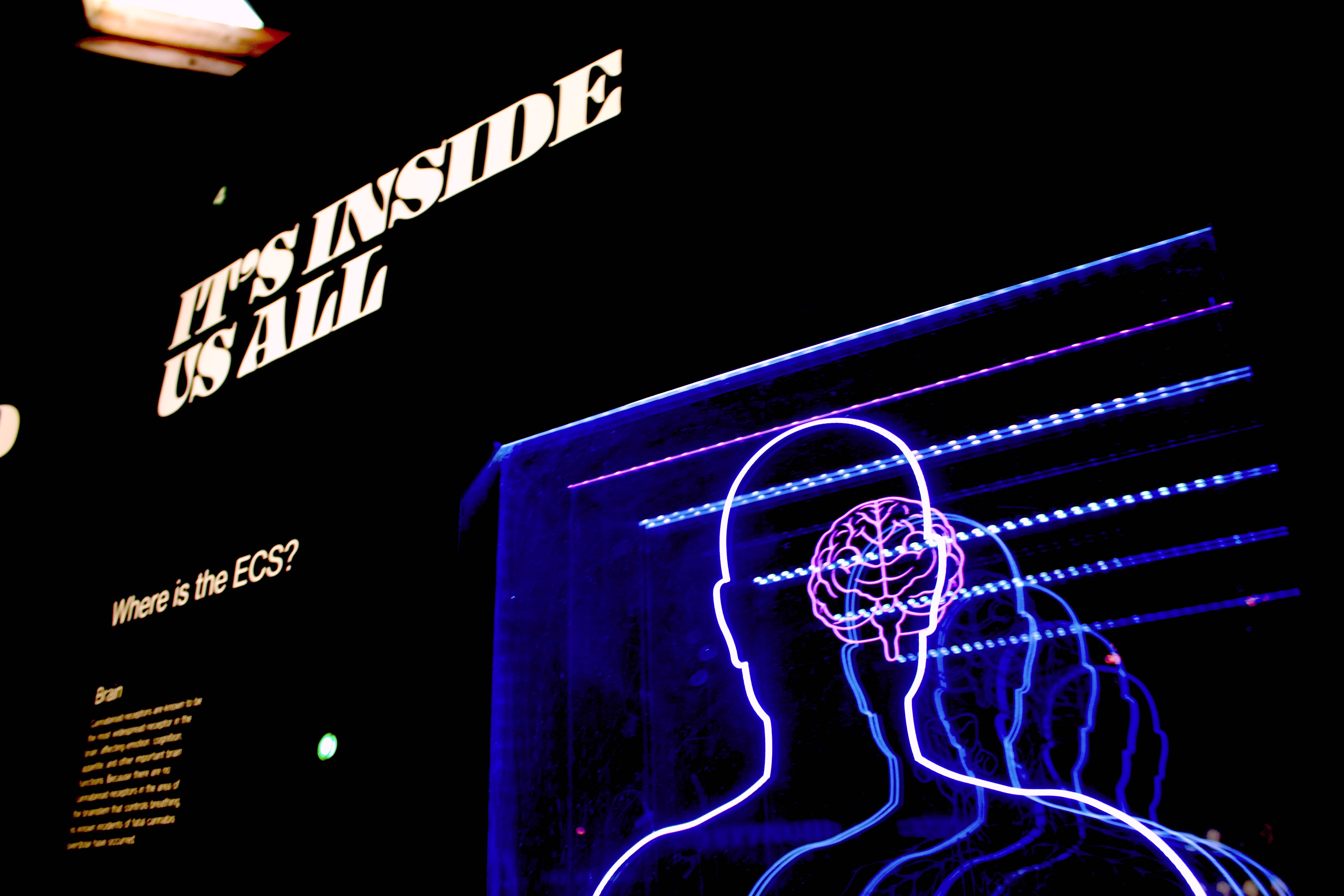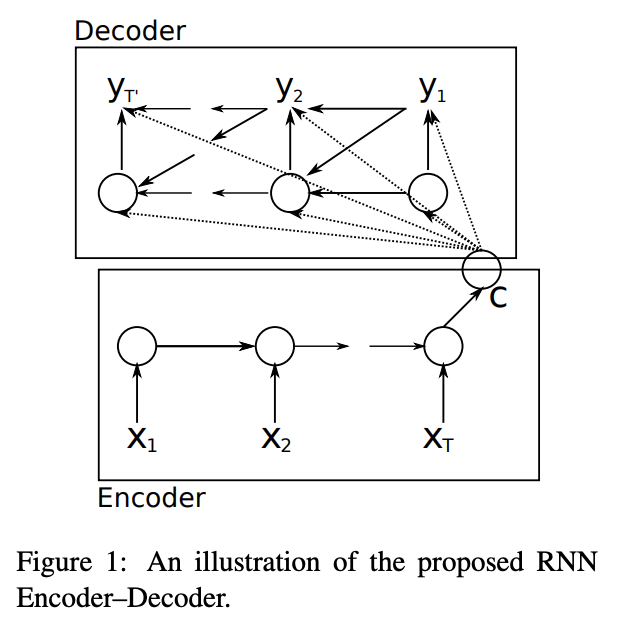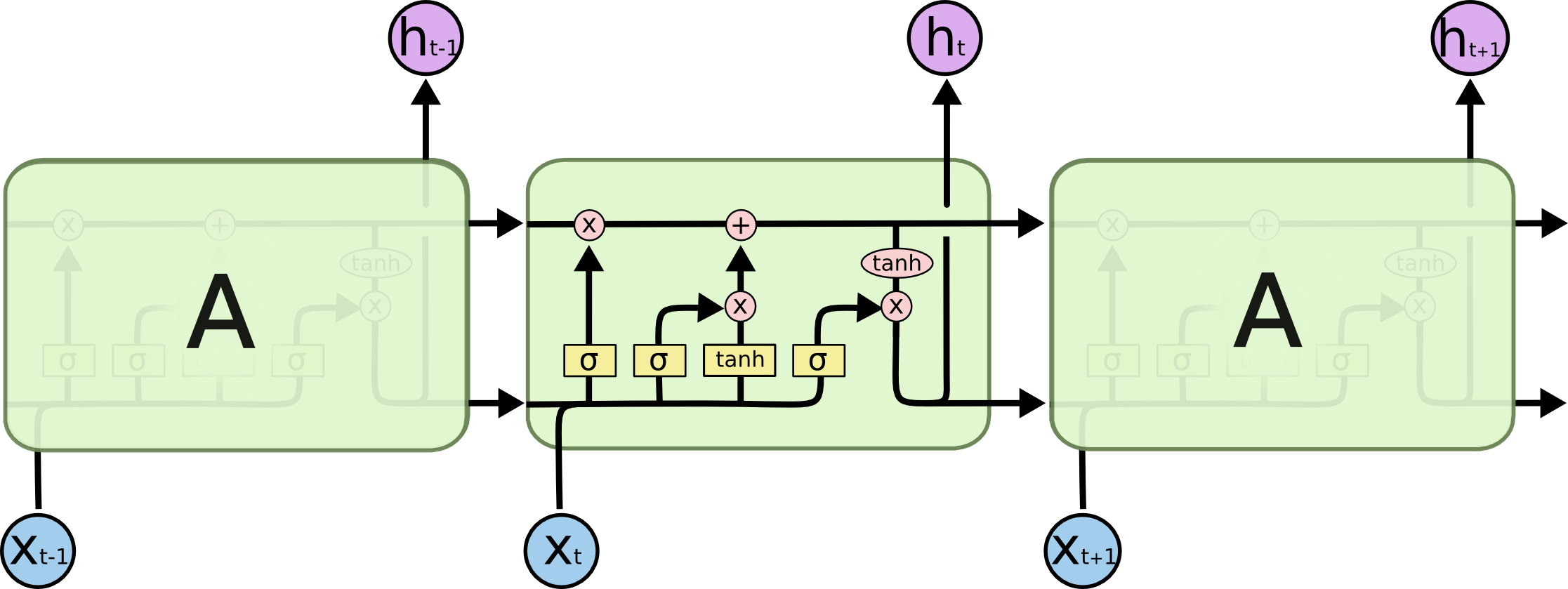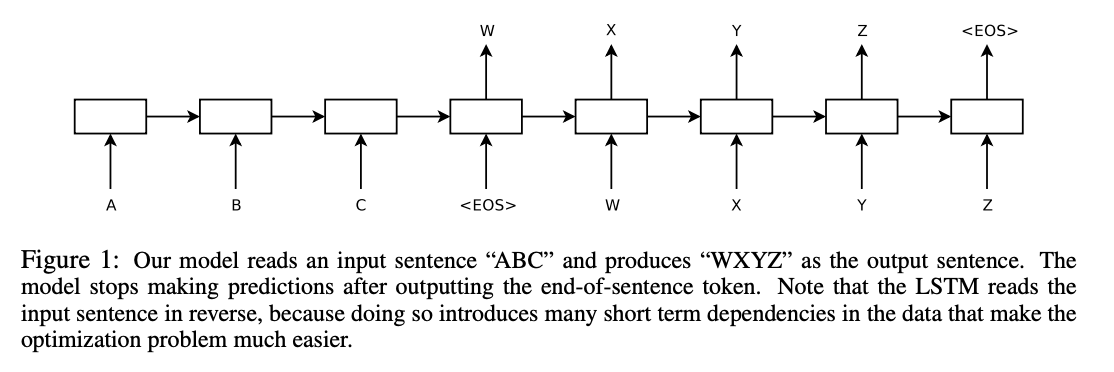|

# Attention Mechanism in Neural Networks - 2. Sequence-to-Sequence (Seq2Seq) (1)

In previous posting, I introduced the attention mechanism and outlined its (not so) short history. In this posting, I will explain the Sequence-to-Sequence (Seq2Seq) architecture, which brought a major breakthrough in neural machine translation and motivated the development of attention.[Image source]

## Motivation - Problem of sequences

Deep neural networks are highly effective tools to model non-linear data for various tasks. It has been proven to be effective in various tasks, e.g., image classification and sentence classification. However, conventional architectures such as multilayer perceptrons are less effective in modeling sequences such as signals and natural language. Therefore, Seq2Seq was proposed to map sequence inputs to sequence outputs. Seq2Seq can process variable-length vectors, mapping them to variable-length vectors.[Photo by Bret Kavanaugh on Unsplash]

Consider the classical application of Seq2Seq to the machine translation task, i.e., translating French sentences (source) to English ones (target). Notice that source sentences have different lengths in terms of words (or characters). The first French sentence “On y va,” which is translated into “Let’s go” in English, has three words or the second, third, and fourth sentences have four, five, and six, respectively. Also, the number of target words are not fixed as well - it can be two to six words in this example.Another potential problem of machine translation is that source (and target) words are often dependent on each other. For instance, when we see the word “I” at the start of the sentence, we are more likely to see “am” as the second word than “are.” Conversely, if we see “You,” we are likely to see “are” than “am.” Thus, it is important to model temporal dependencies among different words (and characters) in a sentence.

Also, source and target words have dependencies between them. In other words, a source word is more likely to be related with some of the target words than others. For instance, “Pour” in the second French sentence is more aligned with “For” in the English sentence and “la” with “the” and so on. This is more deeply considered in Alignment models with attention

## Seq2Seq architecture

Therefore, Seq2Seq was proposed to model variable-length source inputs with temporal dependencies. Cho et al. (2014) is one of the frontier studies investigating neural machine translation with sequences. Their RNN Encoder-Decoder architecture is comprised of two recurrent neural networks - i.e., encoder and decoder.[Image source: Cho et al. (2014)]

Both encoder and decoder comprise multiple recurrent neural network (RNN) cells such as LSTM and GRU cells. The number of cells varies across different instances to take into account varying number of source and target words. Each RNN cells have multiple outputs to model dependencies among input vectors. In addition to sequence outputs, LSTM cells have hidden and cell states and GRU cells have hidden states. For more information on RNN structure, please refer to RNN tutorial with Pytorch.[Image source]

The final hidden state of the encoder, c, functions as a summary of the inputs to the encoder, i.e., the source sentence. In other words, information from the source sentence is distilled in a vector with a fixed dimensionality. In the decoder , c is an input to RNN cells, along with previous hidden state and target word. Therefore, the hidden state at level t is calculated as below (f is the RNN operation in this context).

\begin{equation} h_t = f(h_{t-1}, y_{t-1}, c) \end{equation}

And the output at each step t is the probability of predicting a certain word at that step with the activation function g.

\begin{equation} P(y_t|y_{t-1}, y_{t-2}, …, y_1, c) = g(h_t, y_{t-1}, c) \end{equation}

Then, the calculated probabilities are softmaxed to find the word with the highest predicted probability.[Image source: Sutskever et al. (2014)]

Following Cho et al. (2014), many studies such as Sutskever et al. (2014) proposed similar deep learning architectures to RNN Encoder-Decoder with LSTM. Hence, we call variants of RNN models mapping sequences to sequences with the encoder and decoder Seq2Seq.

In this posting, I introduced Seq2Seq and its overall architecture. In the next posting, I will explain the Seq2Seq architecture in detail, while implementing it with Pytorch. Thank you for reading.